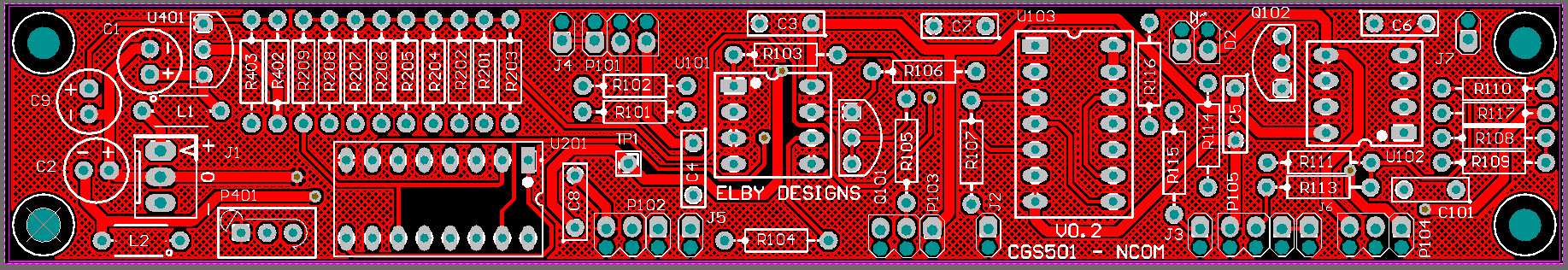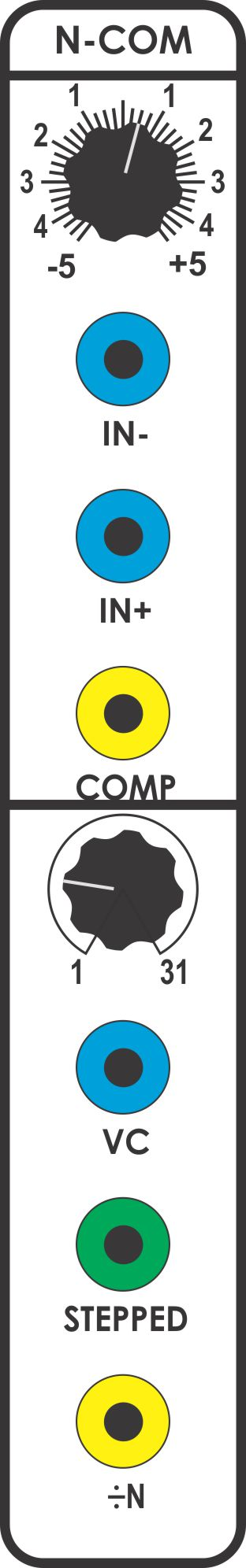CGS501 - N-COMThe Divide-N Comparator consists of two section: a comparator and a voltage-controlled pulse divider. The divider section outputs a pulse once every 'N' comparator pulses, where 'N' is a number from 1 to 31 settable with a control voltage at the dividers [VC] input (or manually via the [1-31] knob). Additionally, the divider outputs a staircase wave with 'N' steps. This will produce whole-tone steps when plugged in to the 1V/Octave input of a VCO.This module has two distinct areas of use (in addition to the normal functions of the comparator):- For audio frequencies, the divider can be set to output sub-divided frequencies with digital precision. Output frequency depends on "N". If "N" = 2, 3, 4 etc the output frequencies will be an octave, an octave and fifth, or two octaves below the input frequency respectively. Because "N" is voltage controllable, arpeggios and various melodies can easily be programmed. The nature of this (integer) division results in frequencies that fall along the sub-harmonic series , a series that has great tonal charm. For sub-audio frequencies, the divider acts like a counter, outputting a pulse only after "N" number of input pulses. Input pulses can be fairly random, or regular. This capability is especially powerful for determining tempos and rhythmic patterns when using several sequencers (especially if the [VC] input is taken from one of the sequencer's rows of controls). In a more random situation, using a microphone preamp/detector  as input, the divider might be set to count how many times a sound or certain loudness will have occurred, and be set to trigger an event upon reaching that count. Since the count can be made variable (from 1 to 31), fairly complex and subtle interactions can be generated.
• PCB info: 1" x 6" with four 3.2mm mounting holes 0.15" in from the edges.
 Component Kit BOM Build Notes Hardware Kit BOM